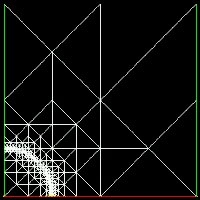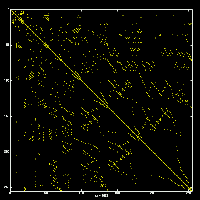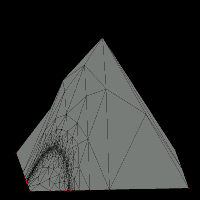MCLite User's Guide

Version 0.1Overview

MCLite is an adaptive finite element MATLAB package for general second-order nonlinear elliptic equations in the plane. MCLite is essentially a 2D MATLAB version of MC, which is an adaptive multilevel finite element code for 2- and 3-manifolds. While MCLite is a MATLAB package, it is not a ``toy'' code; it retains many of the features of MC, and in fact MC development is often prototyped using MCLite first, with later reimplementation in MC. MCLite can handle the same general class of nonlinear elliptic operators that MC was developed for, except that MCLite is restricted to 2-manifolds. As in the case of MC, the MCLite user provides only a nonlinear weak form, the corresponding bilinear linearization form, and a function representing the essential boundary condition. The user also provides a list of triangles representing the polygonal domain 2-manifold. MCLite and MC use the same input and output file formats, so that they can be used collaboratively. While fairly general, MCLite is simple enough to be used as a teaching tool.

Features

MCLite implements piecewise linear simplicial finite elements, a posteriori error estimation, adaptive mesh refinement (quadrasection and bisection), continuation methods, and global inexact-newton methods for nonlinear elliptic equations on arbitrary polyhedral 2-manifold domains. Adaptive mesh refinement is implemented using quadrasection, and/or bisection of triangles by longest edge, and the adaptivity is driven by a user-provided a posteriori error estimator. All of the adaptivity algorithms have linear complexity and are quite fast, thanks to the use of the "ringed vertex" datastructure (borrowed from MC). A minimal Geomview clone called SG can be used with MCLite as an interactive mesh manipulation tool, in place of MATLAB's builtin graphics routines.The MCLite package solves the following general second order nonlinear elliptic equation posed in a weak formulation:

Find [u+g] in H^1_D(Omega) such that (F(u),v) = 0, for all v in H^1_D(Omega),

where (F(u),v) is the nonlinear weak form generated (by appropriate integration by parts) from a second-order, divergence-form, nonlinear elliptic equation in a polyhedral domain in R^2. MCLite discretizes the problem using piecewise linear elements, and solves the resulting nonlinear algebraic equations using a damped Newton iteration. The linear systems are solved using MATLAB's builtin sparse direct solver, although a hook is provided for experimenting with iterative methods. Given the general nature of the above formulation, quite general boundary conditions can be employed. The user must specify the essential boundary condition function g, the domain Omega, the nonlinear form (F(u),v), and also a linearization form (DF(u),v).

MCLite can also be used as an interactive tool for working with MC. For example, MCLite uses the same mesh file format used by MC, and can therefore be used as an interactive tool for constructing, manipulating, and viewing the simplex meshes used by MC (both 2D meshes of triangles and 3D meshes of tetrahedra). MC on the other hand can produce MCLite-format (simply MATLAB sparse-matrix format) output files containing sparse matrices (e.g. the Jacobian at a particular Newton iteration) which can in turn be read in by MCLite and analyzed or manipulated.Obtaining MCLite

MCLite was developed by Michael Holst in 1994 as a teaching tool and also as a prototyping tool for developing MC, MCLite is copylefted and distributed under the GNU General Public License (GPL). (A copy of the GPL should be included with your copy of MCLite.)